#Function Repository Resource:

# ArcSecDegree

Compute the inverse secant of a number and return a result in degrees

Contributed by: Paco Jain (Wolfram Research)
 ResourceFunction["ArcSecDegree"][x] computes the inverse secant of x in degrees.

## Details and Options

ArcSecDegree is a mathematical function, suitable for both symbolic and numerical manipulation.
ArcSecDegree[x] is undefined for x = 0.
ArcSecDegree[x] is undefined in the range -1 to 1.
For x outside the interval -1 to 1, ArcSecDegree[x] always in the range 0 to 180 excluding 90.
For certain special arguments, ArcSecDegree automatically evaluates to exact values.
ArcSecDegree can be evaluated to arbitrary numerical precision.
ArcSecDegree automatically threads over lists.

## Examples

### Basic Examples

Compute the inverse secant of a number:

 In:=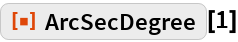Out=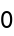Plot over a given range of values:

 In:=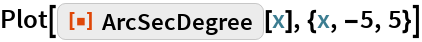Out=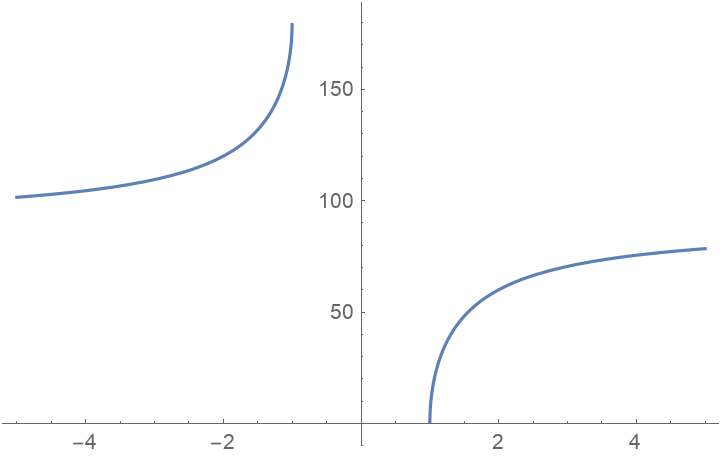### Scope

Evaluate numerically:

 In:=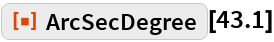Out=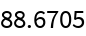Evaluate to high precision:

 In:=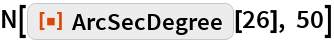Out=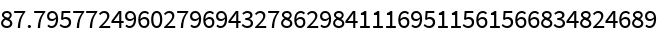## Requirements

Wolfram Language 11.3 (March 2018) or above